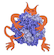IMP  2.4.0 The Integrative Modeling Platform
isd Directory ReferenceDirectory dependency graph for isd:

## Files

file  AmbiguousNOERestraint.h [code]
A lognormal restraint that uses the ISPA model to model NOE-derived distance fit.

file  AmbiguousRestraint.h [code]
An implementation of the d-norm to make an ambiguous restraint.

A pmf based likelihood function with prior knowledge on the flase positive rate.

file  bivariate_functions.h [code]
Classes for general functions.

Normal distribution of Function.

A pmf based likelihood function with prior knowledge on the flase positive rate.

Normal distribution of Function.

A sigmoid shaped restraint between residues with discrete classifier and ambiguous assignment. To be used with cross-linking mass-spectrometry data.

file  em_utilities.h [code]
Common scoring functions.

file  FNormal.h [code]
Normal distribution of Function.

file  FretData.h [code]
Auxiliary class useful for FRET_R restraint.

file  FretRestraint.h [code]
FRET_R restraint to use in vivo FRET data.

file  GaussianEMRestraint.h [code]
Restraint two sets of gaussians (model and gmm derived from EM map)

file  GaussianProcessInterpolation.h [code]
Normal distribution of Function.

file  GaussianProcessInterpolationRestraint.h [code]
kernel::Restraint and ScoreState for GaussianProcessInterpolation

file  GaussianRestraint.h [code]
A lognormal restraint that uses the ISPA model to model NOE-derived distance fit.

file  HybridMonteCarlo.h [code]
A hybrid monte carlo implementation.

file  isd_config.h [code]

file  JeffreysRestraint.h [code]
A restraint on a scale parameter.

file  LognormalRestraint.h [code]
A lognormal restraint that uses the ISPA model to model NOE-derived distance fit.

file  LogWrapper.h [code]
Calculate the -Log of a list of restraints.

file  MarginalHBondRestraint.h [code]
A lognormal restraint that uses the ISPA model to model HBond-derived distance fit.

file  MarginalNOERestraint.h [code]
A lognormal restraint that uses the ISPA model to model NOE-derived distance fit.

file  isd/MolecularDynamics.h [code]
Simple molecular dynamics optimizer.

file  MolecularDynamicsMover.h [code]
A modifier which perturbs XYZs or Nuisances with a constant energy MD simulation.

file  MultivariateFNormalSufficient.h [code]
Normal distribution of Function.

file  NOERestraint.h [code]
A lognormal restraint that uses the ISPA model to model NOE-derived distance fit.

file  Nuisance.h [code]
A decorator for nuisance parameters particles.

file  RepulsiveDistancePairScore.h [code]
A simple quadric repulsive term between two atoms. Restraint is zero when the distance equals the sum of the radii plus the shift.

file  Scale.h [code]
A decorator for scale parameters particles.

file  Switching.h [code]
A decorator for switching parameters particles.

file  TALOSRestraint.h [code]
TALOS dihedral restraint between four particles.

file  UniformPrior.h [code]
A restraint on a scale parameter.

file  univariate_functions.h [code]
Classes for general functions.

file  vonMises.h [code]
Normal distribution of Function.

file  vonMisesKappaConjugateRestraint.h [code]
Conjugate prior for $$\kappa$$ in the von Mises distribution.

file  vonMisesKappaJeffreysRestraint.h [code]
Jeffreys prior for $$\kappa$$ in the von Mises distribution.

file  vonMisesSufficient.h [code]
Normal distribution of Function.

file  Weight.h [code]
Add weights for a set of states to a particle.

file  WeightMover.h [code]
A mover that transforms a rigid body.

file  WeightRestraint.h [code]
Put description here.